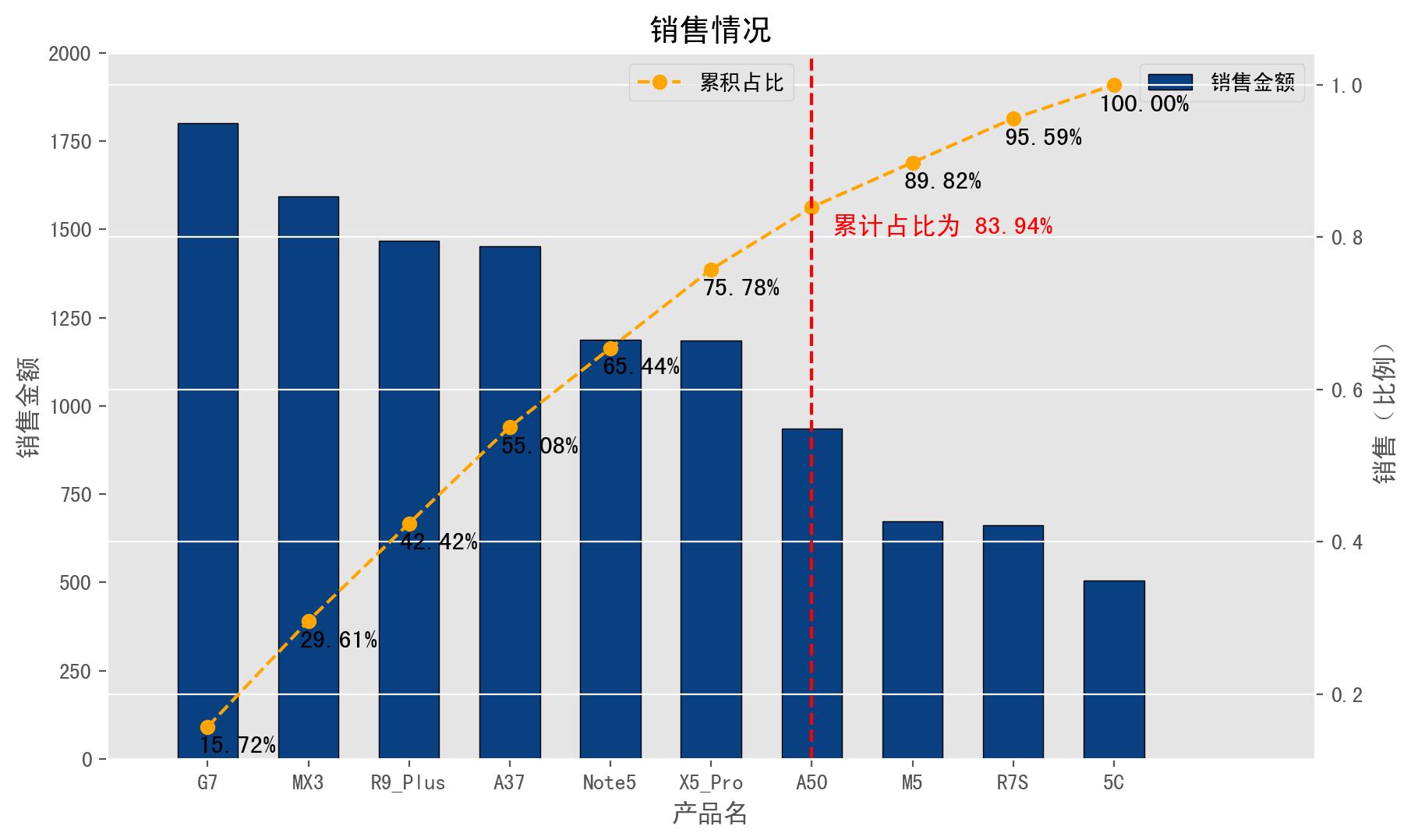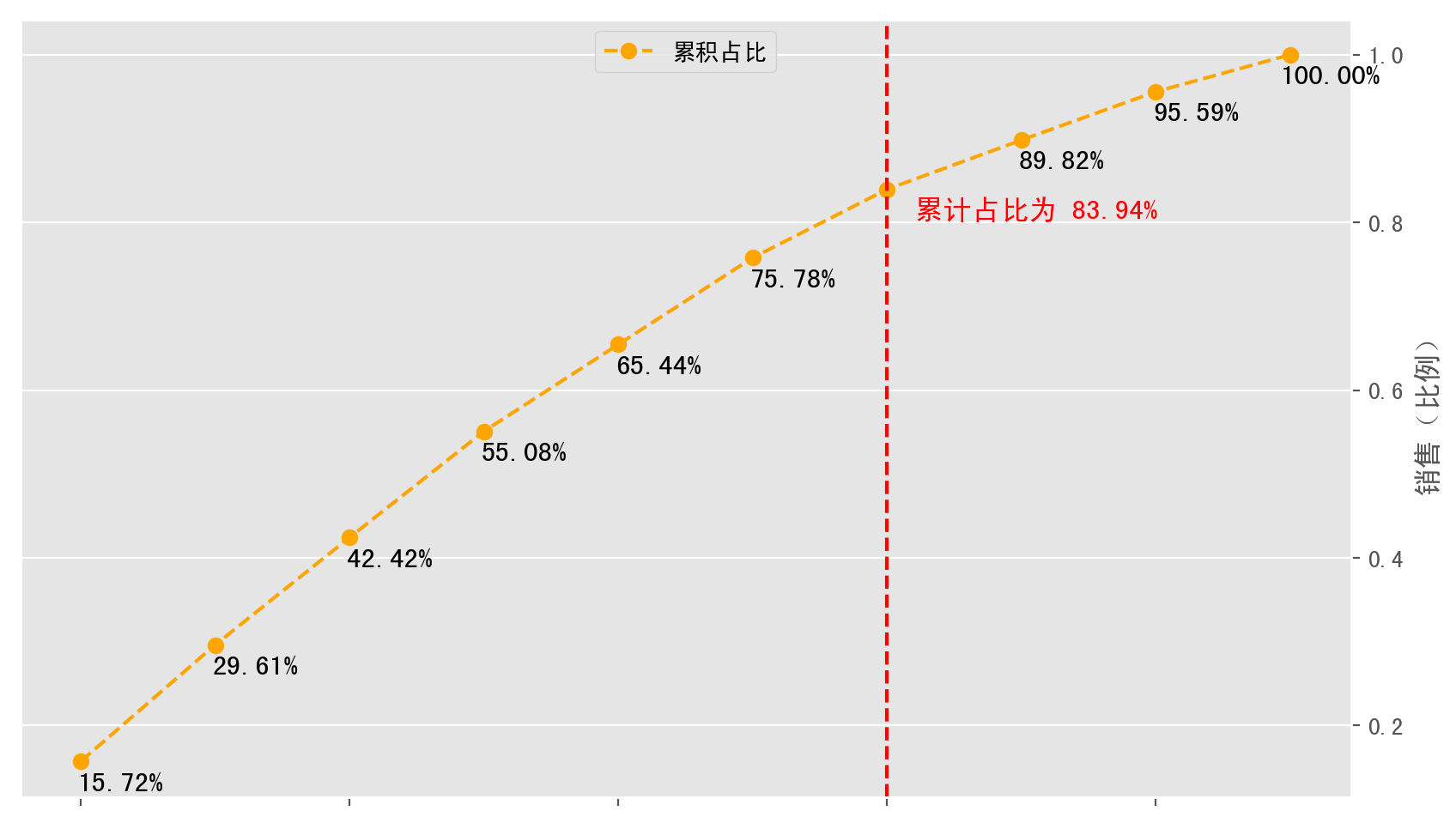# Anders Wangimport numpy as np
import pandas as pd
import matplotlib.pyplot as plt

%matplotlib inline
%config InlineBackend.figure_format = 'retina'

# 创建模拟数据，10个品类产品的销售额
data = pd.Series(np.random.randn(10)*500+1000,
index=['A37','A50','R7S','Note5',
'G7','R9_Plus','5C','X5_Pro',
'MX3','M5'])
print(data)


A37        1784.729567
A50         198.971079
R7S         985.258417
Note5      1348.619119
G7         1330.237773
R9_Plus     188.307306
5C         2105.157302
X5_Pro     1225.447782
MX3         957.038300
M5         1346.797999
dtype: float64


# 将数值按照从大到小排序
data.sort_values(ascending=False, inplace=True)
# 构建画布
plt.figure(figsize=(10,6))

# 先构建一个从大到小排列的，标准柱状图。
data.plot(kind='bar', colormap='GnBu_r', width=0.6, edgecolor='black', rot=0)

plt.xlim(-1,11)
plt.ylim(0,2000)

plt.xlabel('产品名')
plt.ylabel('销售金额')
plt.title('销售情况')
plt.legend(['销售金额'], loc='upper right')# 累加值 除以 总数值 得到每个阶段累加值的占比值。
proportion = data.cumsum() / data.sum()

# 用布尔索引列出大于等于0.8的数值列表，而列表里第一行就是大于80%的关键点，我们取得它的索引名称。
key = proportion[proportion >= 0.8].index

# 根据之前得到的索引名称，就可以从data数据中得到该名称的索引位置。
key_number = data.index.tolist().index(key)

proportion.plot(style='--ko', secondary_y=True, color='#FFA500')

plt.axvline(key_number, linestyle='--', color='red')

for x, y, s in zip(range(len(data)), proportion.values, proportion):
if x == key_number:
plt.text(key_num+0.2, y-0.035, '累计占比为 {:.2%}'.format(s), color='red', fontsize=12)
else:
plt.text(x+0.3, y-0.035, "{:.2%}".format(s), fontsize=12, horizontalalignment='center', color='black')

plt.ylabel('销售（比例）')
plt.legend(['累积占比'], loc='upper center')import numpy as np
import pandas as pd
import matplotlib.pyplot as plt

%matplotlib inline
%config InlineBackend.figure_format = 'retina'

# 创建模拟数据，10个品类产品的销售额
data = pd.Series(np.random.randn(10)*500+1000,
index=['A37','A50','R7S','Note5',
'G7','R9_Plus','5C','X5_Pro',
'MX3','M5'])

# 将数值按照从大到小排序
data.sort_values(ascending=False, inplace=True)
# 构建画布
plt.figure(figsize=(10,6))

# 先构建一个从大到小排列的，标准柱状图。
data.plot(kind='bar', colormap='GnBu_r', width=0.6, edgecolor='black', rot=0)
plt.xlim(-1,11)
plt.ylim(0,2000)
plt.xlabel('产品名')
plt.ylabel('销售金额')
plt.title('销售情况')
plt.legend(['销售金额'], loc='upper right')

# 累加值 除以 总数值 得到每个阶段累加值的占比值。
proportion = data.cumsum() / data.sum()

# 用布尔索引列出大于等于0.8的数值列表，而列表里第一行就是大于80%的关键点，我们取得它的索引名称。
key = proportion[proportion >= 0.8].index

# 根据之前得到的索引名称，就可以从data数据中得到该名称的索引位置。
key_number = data.index.tolist().index(key)

proportion.plot(style='--ko', secondary_y=True, color='#FFA500')

plt.axvline(key_number, linestyle='--', color='red')

for x, y, s in zip(range(len(data)), proportion.values, proportion):
if x == key_number:
plt.text(key_num+0.2, y-0.035, '累计占比为 {:.2%}'.format(s), color='red', fontsize=12)
else:
plt.text(x+0.3, y-0.035, "{:.2%}".format(s), fontsize=12, horizontalalignment='center', color='black')

plt.ylabel('销售（比例）')
plt.legend(['累积占比'], loc='upper center')


## 用yield关键字创建生成器

Python使用 生成器(generator) 对延迟操作提供了支持。所谓延迟操作，是指在需要的时候才产生结果，而不是立即产生结果，因此它不会在内存中创建和存储整个序列，这也是生成器的主要好处。 什么…